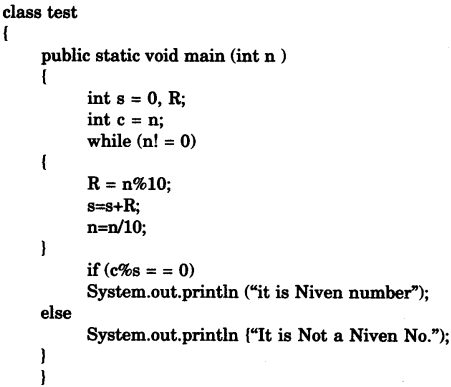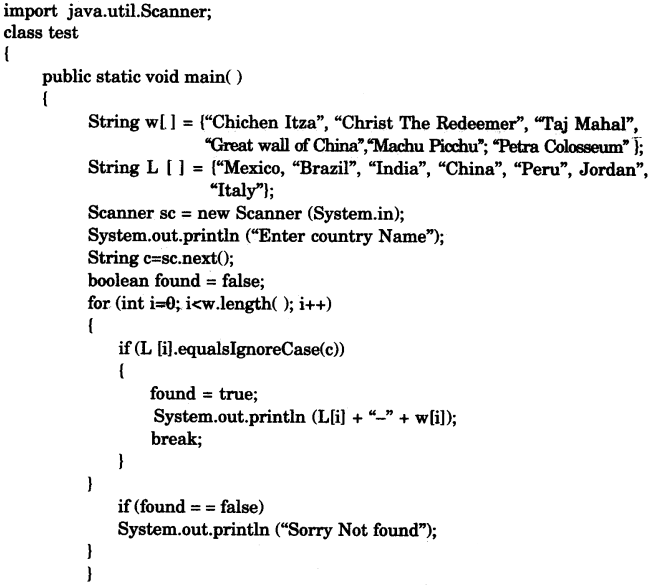## ICSE Computer Applications Previous Year Question Paper 2016 Solved for Class 10

ICSE Paper 2016
COMPUTER APPLICATIONS

(Two Hours)
Answers to this Paper must be written on the paper provided separately.
You will not be allowed to write during the first 15 minutes.
This time is to be spent in reading the question paper.
The time given at the head of this Paper is the time allowed for writing the answers.
This paper is divided into two Sections.
Attempt all questions from Section A and any four questions from Section B.
The intended marks for questions or parts of questions are given in brackets [ ].

Section ‘A’ (40 Marks)

(Attempt all questions)

Question 1:
(a) Define Encapsulation. 
(b) What are keywords ? Give an example. 
(c) Name any two library packages. 
(d) Name the type of error ( syntax, runtime or logical error) in each case given below: 
(i) Math.sqrt (36 – 45)
(ii) int a;b;c;
(e) If int x [ ] = { 4, 3,7, 8, 9,10}; what are the values of p and q ? 
(i) p = x.length
(ii) q = x + x * x

(a) Encapsulation: It is process of wrapping code and data together into a single unit (called class).

(b) Keywords: Keywords are reserved words in Java, which have a special meaning in the language.
Example: if, void, int etc.

(c) (i) java.io
(ii) java.util

(d) (i) logical error
(ii) syntax error

(e) (i) Value of P will be 6.
(ii) q = x + x * x
= 7 + 10 * 3
= 7 + 30 = 37
value of q is 37.

Question 2:
(a) State the difference between == operator and equals ( ) method. 
(b) What are the types of casting shown by the following examples: 
(i) char c = (char) 120;
(ii) int x = ‘t’;
(c) Differentiate between formal parameter and actual parameter. 
(d) Write a function prototype of the following: 
A function PosChar which takes a string argument and a character argument and returns an integer value.
(e) Name any two types of access specifiers. 

(a)

 = = operator equals ( ) method It is a relational operator. It is a method of string class. It checks for equality of primitive type of values. It checks for equality of two string type of values.

(b) (i) Explicit type conversion,
(ii) Implicit type conversion.

(c)

 Formal parameter Actual parameter They are variables define in func­tion signature. They are values given to the function at the time of function call. They receive value from actual parameter. They give values to formal parameter.

(d) int PosChar (String str, char ch)

(e) Two types of access specifiers:
1. public
2. . private

Question 3:
(a) Give the output of the following string functions: 
(i) “MISSISSIPPI”.indexOf(‘S’) + “MISSISSIPPF”.lastIndexOf(‘I’)

(b) Give the output of the following Math functions: 
(i) Math.ceil(4.2)
(ii) Math.abs(-4)

(c) What is a parameterized constructor ? 

(d) Write down java expression for: 
$$T=\sqrt { { A }^{ 2 }+{ B }^{ 2 }+{ C }^{ 2 } }$$

(e) Rewrite the following using ternary operator: 
if (x%2 == o)
System.out.print(“EVEN”);
else
System.out.print(“ODD”);

(f) Convert the following while loop to the corresponding for loop: 
int m = 5, n = 10;
while (n>=1)
{
System.out.println(m*n);
n-;
}

(g) Write one difference between primitive data types and composite data types. 

(h) Analyze the given program segment and answer the following questions: 
(i) Write the output of the program segment.
(ii) How many times does the body of the loop gets executed ?(i) Give the output of the following expression: 
a+= a++ + ++a + – – a + a – – ; when a = 7

(j) Write the return type of the following library functions: 
(i) isLetterOrDigit(char)
(ii) replace(char, char)

(a) (i) 2 + 10 = 12
(ii) -3

(b) (i) 5.0
(ii) 4

(c) Parameterized Constructor: It is a constructor which accepts parameters. When an object is declared using parameterized constructor, the initial values have to be passed as arguments to the constructor.

(d) T = Math.sqrt (A*A + B*B + C*C);

(e) String k;
k = x%2 == 0 ? “EVEN” : “ODD”;
System.out.print(k);

(f) for (int m=5, n=10; n>=1; n – – )
{
System.out.println (m*n);
}

(g)

 Primitive data types Composite data types They are predefined/inbuilt data types. These data types are defined by user and made-up of primitive data type values. Examples : int, byte, long, short, char, float, double, boolean. Examples : Array, class, interface.

(h) (i) Output:
5
10
(ii) 3 times

(i) a = a+ a++ + ++a + – – a + a – – ;
= 7 + 7 + 9 + 8 + 8 = 39

(j) (i) boolean (ii) String

Section B (60 Marks)

Attempt any four questions from this Section.
The answers in this Section should consist of the Programs in either Blue J , environment or any program environment with Java as the base.
Each program should be written using Variable descriptions I Mnemonic Codes so that the logic of the program is clearly depicted.
Flow-Charts and Algorithms are not required.

Question 4:
Define a class named BookFair with the following description: 
Instance variables/Data members :
String Bname — stores the name of the book
double price — stores the price of the book Member methods :
(i) BookFair() — Default constructor to initialize data members
(ii) void Input() — To input and store the name and the price of the book.
(iii) void calculate() — To calculate the price after discount. Discount is calculated based on the following criteria.

 Price Discount Less than or equal to Rs. 1000 2% of price More than Rs. 1000 and less than or equal to Rs. 3000 10% of price More than % 3000 15% of price

(iv) void display() — To display the name and price of the book after discount. Write a main method to create an object of the class and call the above member methods.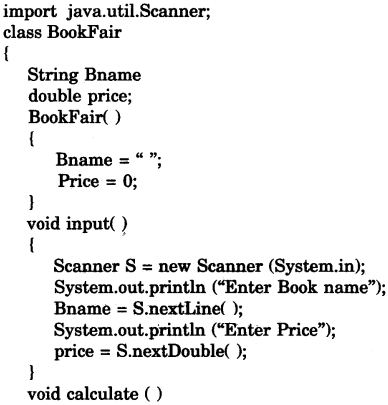Question 5:
Using the switch statement, write a menu driven program for the following: 
(i) To print the Floyd’s triangle [Given below]

1

2      3

4      5       6

7      8       9      10

11    12    13     14    15

(ii) To display the following pattern:

I

I     C

I     C      S

I     C      S     E

For an incorrect option, an appropriate error message should be displayed.Question 6:
Special words are those words which starts and ends with the same letter. 
Examples:
EXISTENCE
COMIC
WINDOW
Palindrome words are those words which read the same from left to right and vice versa
Examples:
MALAYALAM
LEVEL
ROTATOR
CIVIC
All palindromes are special words, but all special words are not palindromes. Write a program to accept a word check and print Whether the word is a palindrome or only special word.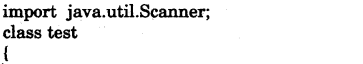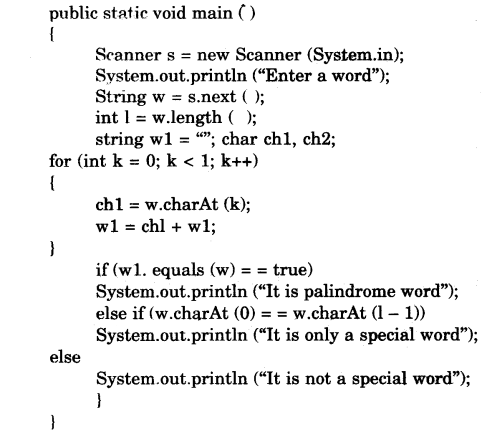Question 7:
Design n class to overload a function SumSeriesO as follows: 
(i) void SumSeries(int n, double x) – with one integer argument and one double argument to find and display the sum of the series given below: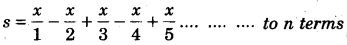(ii) void SumSeries() – To find and display the sum of the following series:
s = 1 + (1 x 2) + (1 x 2 x 3) + ….. + (1 x 2 x 3 x 4 x 20)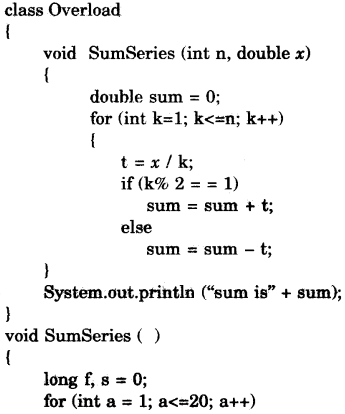Question 8:
Write a program to accept a number and check and display whether it is a Niven number or not. 
(Niven number is that number which is divisible by its sum of digits).
Example:
Consider the number 126.
Sum of its digits is 1+2+6 = 9 and 126 is divisible by 9.